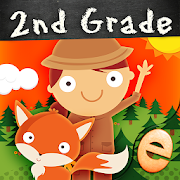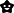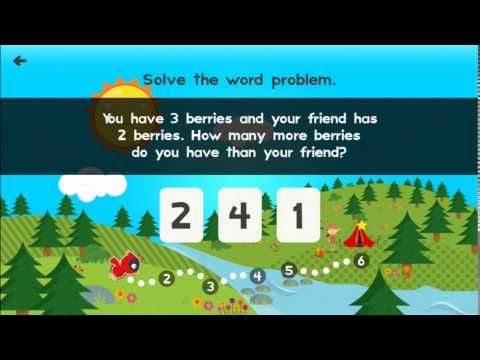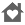# Animal Math Second Grade Math Games for Kids MathEveryoneAges 6-8
8Join Emma in an all new adventure as she helps Oleg the Owl, Chloe the Fox, and all her animal friends to complete over 100 second grade math challenges and find their way through the forest!

This app encourages the cultivation of second grade math skills and adheres to Common Core Standards for second grade math.

SIGNS & OPERATIONS
• Plus, minus, greater than, less than, equal to
• Select which sign is missing from an equation
• Choose the correct operation for a word problem
• Even & odd numbers

ALGEBRAIC THINKING
• Number sequences & patterns
• Equations & word problems within 100
• Determine the unknown number in an equation
• True or false equations

PLACE VALUE
• Counting by 1s, 10s and 100s
• Addition and subtraction by 1s, 10s and 100s
• Find the missing number in a hundreds table
• Greater than or less than within 1000

• Professionally narrated instructions & feedback
• Players are rewarded with positive encouragement
• Parental controls for music, sound & more
• We do not collect personal information period

COMMON CORE STANDARDS
CCSS.MATH.CONTENT.2.OA.A.1 - Use addition and subtraction within 100 to solve one- and two-step word problems.
CCSS.MATH.CONTENT.2.OA.B.2 - Fluently add and subtract within 20
CCSS.MATH.CONTENT.2.OA.C.3 - Determine whether a group of objects (up to 20) has an odd or even number of members
CCSS.MATH.CONTENT.2.OA.C.4 - Use addition to find the total number of objects arranged in rectangular arrays with up to 5 rows and up to 5 columns
CCSS.MATH.CONTENT.2.NBT.A.1 - Understand that the three digits of a three-digit number represent amounts of hundreds, tens, and ones
CCSS.MATH.CONTENT.2.NBT.A.1.A - 100 can be thought of as a bundle of ten ten
CCSS.MATH.CONTENT.2.NBT.A.1.B - The numbers 100, 200, 300, 400, 500, 600, 700, 800, 900 refer to one, two, three, four, five, six, seven, eight, or nine hundreds
CCSS.MATH.CONTENT.2.NBT.A.2 - Count within 1000; skip-count by 5s, 10s, and 100s
CCSS.MATH.CONTENT.2.NBT.A.4 - Compare two three-digit numbers
CCSS.MATH.CONTENT.2.NBT.B.5 - Fluently add and subtract within 100
CCSS.MATH.CONTENT.2.NBT.B.6 - Add up to four two-digit numbers
CCSS.MATH.CONTENT.2.NBT.B.7 - Add and subtract within 1000
CCSS.MATH.CONTENT.2.NBT.B.8 - Mentally add 10 or 100 to a given number 100-900, and mentally subtract 10 or 100 from a given number 100-900
Collapse

Review Policy
4.2
8 total
5
4
3
2
1

## What's New

Performance Improvements

If you love our app, please rate or review it. Thank you!
Collapse

Eligible for Family LibraryLearn More
Updated
August 24, 2019
Size
35M
Installs
500+
Current Version
2.3.0
Requires Android
4.0.3 and up
Content Rating
Everyone
Permissions
Offered By
Eggroll Games
Developer
Eggroll Games LLC 1820 S. Main St. Winston-Salem, NC 27127